# BT

The BT package implements (Adaptive) Boosting Tree for Poisson distributed response variables, using log-link function. When presented with data, the BT package offers the user the ability to build predictive models and explore the influence of different variables on the response, akin to a data mining or exploration task. The built package is based on the original idea proposed by D. Hainaut, J. Trufin and M. Denuit. For more theoretical details, we refer to the following references:

• M. Denuit, D. Hainaut and J. Trufin (2019). Effective Statistical Learning Methods for Actuaries |: GLMs and Extensions, Springer Actuarial.
• M. Denuit, D. Hainaut and J. Trufin (2019). Effective Statistical Learning Methods for Actuaries ||: Tree-Based Methods and Extensions, Springer Actuarial.
• M. Denuit, D. Hainaut and J. Trufin (2019). Effective Statistical Learning Methods for Actuaries |||: Neural Networks and Extensions, Springer Actuarial.
• M. Denuit, D. Hainaut and J. Trufin (2022). Response versus gradient boosting trees, GLMs and neural networks under Tweedie loss and log-link, Accepted for publication in Scandinavian Actuarial Journal.
• M. Denuit, J. Huyghe and J. Trufin (2022). Boosting cost-complexity pruned trees on Tweedie responses: The ABT machine for insurance ratemaking. Paper submitted for publication.
• M. Denuit, J. Trufin and T. Verdebout (2022). Boosting on the responses with Tweedie loss functions. Paper submitted for publication.

To get started with the package a user must:

• have a dataset in a data.frame.
• set the appropriate parameters for the BT model.

Once these steps have been completed, a user can fit their BT model by a call to BT and subsequently: evaluate its performance, make predictions, fit additional trees and plot the relative influence of the various predictor variables

library(BT)

## Define a database

To demonstrate the BT usage, we’ll use a simulated database where the response variable is Poisson distributed. The built database contains $$n = 50000$$ records as well as the following simulated variables (with their interpretation in an insurance MTPL context):

• Y, the Poisson distributed response variable (e.g. the number of claims).
• Gender, Age and Sport which are directly linked to the response variable (e.g. resp. the policyholder’s gender and age, the vehicle type).
• Split which is not linked to the response variable.
• ExpoR which corresponds to the time-exposure (e.g. the well-known exposure-to-risk).
• Based on these variables, we define the rate as Y_normalize = Y/ExpoR.
set.seed(4)
n <- 50000

Gender <- factor(sample(c("male","female"),n,replace=TRUE))
Age <- sample(c(18:65),n,replace=TRUE)
Split <- factor(sample(c("yes","no"),n,replace=TRUE))
Sport <- factor(sample(c("yes","no"),n,replace=TRUE))

lambda <- 0.1*ifelse(Gender=="male",1.1,1)
lambda <- lambda*(1+1/(Age-17)^0.5)
lambda <- lambda*ifelse(Sport=="yes",1.15,1)

ExpoR <- runif(n)

Y <- rpois(n, ExpoR*lambda)
Y_normalized <- Y/ExpoR

dataset <- data.frame(Y,Gender,Age,Split,Sport,ExpoR, Y_normalized)

## Define the BT parameters

Once the database has been imported, the user needs to define the different parameters that will be passed to the algorithm. In this case, the parameters should not be stored upfront in a specific object and a simple call using the argument can be made. We list below all the available parameters:

• formula: a symbolic description of the model to be fit. We emphasize that the offset are not supported with out approach.
• data: the database on which the computations will be performed.
• tweedie.power: Experimental parameter : the Tweedie power referencing to the response variable distribution. Always set to 1 referring to Poisson distribution.
• ABT: bool value to define whether we fit a Boosting Tree (=FALSE) or an Adaptive Boosting Tree (=TRUE).
• n.iter: the number of iterations to user in the fitting.
• train.fraction: the percentage of the data that will be used as training set. The remaining part will be used as validation set.
• interaction.depth: the maximum number of splits in a tree present in the expansion.
• shrinkage: acts as regularization for additional iterations - the smaller the shrinkage generally the better the performance of the fit. However, smaller shrinkage implies that the number of trees may need to be increased to achieve a certain performance.
• bag.fraction: the fraction of the training observations randomly sub-sampled to fit a tree in each iteration. This has the effect of reducing the variance of the boosted model.
• colsample.bytree: the number of variables randomly sampled that will be used to build the next tree in the expansion.
• keep.data: allows the user the save the different databases used during the algorithm in a BTData object.
• is.verbose: whether the algorithm evolution has to be printed out.
• cv.folds: the number of cross-validation folds to create. If set to 1 (by default), no cross-validation is performed.
• folds.id: used in case of cross-validation. If defined, allows the user to specify in what fold each observation belongs. If cv.folds is greater than 1 and folds.id is well defined, the latter will take over.
• n.cores: in case of cross-validation, the number of cores used to perform the parallelization. Please note that in the cross-validation context, a call to the parLapply function is made (whatever the number of cores). This parameter is originally set to cv.folds.
• tree.control: the proposed algorithm is based on the rpart package. This parameter will be used to originally build each tree in the expansion. We emphasize that if the interaction.depth is set to NULL, each tree in the expansion will be built thanks to this parameters with no further treatment. We recommend this option for advanced user only.
• weights: a vector representing the weight given to each observation. By default, each observation as the same weight (=1).
• seed: some of the parameters bring randomness during the algorithm. This parameter allows the user to replicate the results.

We emphasize that performing a cross-validation will produce a first global model trained on the full training set as well as different cv related BT models. The former will generally further be used while the later will help to e.g. determine the performances.

## Application to the created database

Let us now build the first model. Let us note/suppose that:

• As already mentioned, the simulated response variable Y follows a Poisson distribution. This first step can normally be achieved thanks to a short database analysis.
• The user wants to use all available explanatory variables to explain the response variable Y. In usual MTPL pricing, the ExpoR variable allows to consider the time-exposure to risk (e.g. the Policyholder contract’s duration). The latter variable is thus generally set in offset. In a Tweedie framework with log-link function, we emphasize that working with offset is equivalent to build a model on the rate response variable and adjusted weights. More precisely, the variable Y_normalized will be used as response variables with weights given by ExpoR (instead of original values, i.e. 1). This choice was made during modelling by the author.
• We’ll work in the context of Boosting Tree and perform 300 iterations (=n.iter).
• The interaction.depth and shrinkage parameters will respectively be set to 4 and 0.01.
• The train.fraction will be set to 0.8, meaning that the first 0.8*nrow(data) will be considered as training set and the remaining data records as validation set. Moreover, random effects such as bagging and columns sample for each tree will be defined. The bag.fraction and colsample.bytree parameters will respectively be set to 0.5 and 3.
• 3-cv will be performed for this example.
BT_algo <- BT(formula = as.formula("Y_normalized ~ Age + Sport + Split + Gender"),
data = dataset,
ABT = F,
n.iter = 300,
train.fraction = 0.8,
interaction.depth = 4,
shrinkage = 0.01,
bag.fraction = 0.5,
colsample.bytree = 3,
keep.data = T,
is.verbose = F,
cv.folds = 3,
folds.id = NULL,
n.cores = 1,
weights = ExpoR,
seed = 44)

Now that the first fit is made, we’ll focus on the different results/functions that are available within the package.

### BT outputs

Multiple results are stored in the BT_algo run (namely, a BTFit object)

First of all, almost all the parameters that have been used during the call are stored in the result object:

# 'Fitting parameters
BT_algo$call ## BT(formula = as.formula("Y_normalized ~ Age + Sport + Split + Gender"), ## data = dataset, ABT = F, n.iter = 300, train.fraction = 0.8, ## interaction.depth = 4, shrinkage = 0.01, bag.fraction = 0.5, ## colsample.bytree = 3, keep.data = T, is.verbose = F, cv.folds = 3, ## folds.id = NULL, n.cores = 1, weights = ExpoR, seed = 44) BT_algo$distribution
##  1
BT_algo$BTParams ##$ABT
##  FALSE
##
## $train.fraction ##  0.8 ## ##$shrinkage
##  0.01
##
## $interaction.depth ##  4 ## ##$bag.fraction
##  0.5
##
## $n.iter ##  300 ## ##$colsample.bytree
##  3
##
## $tree.control ##$tree.control$minsplit ##  2 ## ##$tree.control$minbucket ##  1 ## ##$tree.control$cp ##  -Inf ## ##$tree.control$maxcompete ##  4 ## ##$tree.control$maxsurrogate ##  5 ## ##$tree.control$usesurrogate ##  2 ## ##$tree.control$surrogatestyle ##  0 ## ##$tree.control$maxdepth ##  4 ## ##$tree.control$xval ##  0 ## ## ## attr(,"class") ##  "BTParams" BT_algo$keep.data
##  TRUE
BT_algo$is.verbose ##  FALSE BT_algo$seed
##  44
BT_algo$cv.folds # #used folds ##  3 head(BT_algo$folds, 10) # folds created.
##   2 2 1 1 3 3 3 1 3 1
# Name of weights, response and explnatory variables used
BT_algo$w ##  "w" BT_algo$response
##  "Y_normalized"
BT_algo$var.name ##  "Age" "Sport" "Split" "Gender" One can then continue by having a look at the initialization that is performed as well as the related errors: # Tweedie with intercept only. BT_algo$BTInit$initFit  ##  0.1480054 # Init training error BT_algo$BTInit$training.error ##  0.3685759 # Init validation error BT_algo$BTInit$validation.error ##  0.3528488 If keep.data = TRUE, the created training and validation set are also returned: head(BT_algo$BTData$training.set) ## Y_normalized Age Sport Split Gender w currTrainScore ## 1 0 28 yes no female 0.10197573 -1.878222 ## 2 0 62 no yes male 0.71179081 -2.021452 ## 3 0 37 no no male 0.37298030 -1.915680 ## 4 0 56 yes no male 0.62134765 -1.895872 ## 5 0 36 yes no male 0.10604182 -1.846164 ## 6 0 24 yes yes female 0.05905927 -1.866964 head(BT_algo$BTData$validation.set, 5) ## Y_normalized Age Sport Split Gender w currValScore ## 40001 0.00000 49 yes no female 0.9998001 -1.876190 ## 40002 0.00000 39 no yes male 0.7711231 -1.991724 ## 40003 0.00000 29 yes yes male 0.1610786 -1.834477 ## 40004 0.00000 33 no yes female 0.5680789 -2.038986 ## 40005 2.98261 40 yes no female 0.3352768 -1.895784 One can also have a look at the fitted values (on the score scale) as well as the errors computed across the different iterations (on training set, validation set, the OOB improvement and the cross-validation error): # Obtained fitting results at the end - Full BT and CV. head(BT_algo$fitted.values)
##  -1.878222 -2.021452 -1.915680 -1.895872 -1.846164 -1.866964
head(BT_algo$cv.fitted) # cv fitted. ##  -1.892785 -1.934626 -1.995363 -1.854360 -1.845289 -1.889145 # Errors computed. head(BT_algo$BTErrors$training.error) ##  0.3637171 0.3691590 0.3623403 0.3644938 0.3650795 0.3699250 head(BT_algo$BTErrors$validation.error) ##  0.3528371 0.3528110 0.3528009 0.3527770 0.3527524 0.3527456 head(BT_algo$BTErrors$oob.improvement) ##  2.336664e-05 3.498181e-05 9.592938e-06 2.194806e-05 2.780747e-05 ##  1.158397e-05 head(BT_algo$BTErrors$cv.error) ##  0.3685543 0.3685281 0.3685146 0.3684967 0.3684822 0.3684594 Finally, we note that all the trees fitted during the algorithm are stored in the BTIndivFits list: BT_algo$BTIndivFits[] # First tree in the expansion
## n= 20000
##
## node), split, n, deviance, yval
##       * denotes terminal node
##
##  1) root 20000 7274.6970 0.9851696
##    2) Age>=18.5 19600 7038.6580 0.9661252
##      4) Age>=38.5 11193 3896.8360 0.9131932 *
##      5) Age< 38.5 8407 3136.2120 1.0370160
##       10) Gender=female 4225 1474.3530 0.9393184
##         20) Age>=19.5 4026 1380.0720 0.9104576 *
##         21) Age< 19.5 199   89.4214 1.5041700 *
##       11) Gender=male 4182 1656.0760 1.1354260 *
##    3) Age< 18.5 400  215.7846 1.8509420 *
# One can also access to the usual tree outputs.
head(BT_algo$BTIndivFits[]$frame)
##       var     n        wt      dev      yval   complexity ncompete nsurrogate
## 1     Age 20000 1478.9331 7274.697 0.9851696 0.0027842524        2          0
## 2     Age 19600 1448.0725 7038.658 0.9661252 0.0007830279        2          0
## 4  <leaf> 11193  829.0393 3896.836 0.9131932 0.0007353703        0          0
## 5  Gender  8407  619.0332 3136.212 1.0370160 0.0007830279        2          2
## 10    Age  4225  310.9132 1474.353 0.9393184 0.0006680935        1          0
## 20 <leaf>  4026  296.6375 1380.072 0.9104576         -Inf        0          0
##         yval2.1      yval2.2
## 1     0.9851696 1457.0000000
## 2     0.9661252 1399.0000000
## 4     0.9131932  757.0000000
## 5     1.0370160  642.0000000
## 10    0.9393184  292.0000000
## 20    0.9104576  270.0000000

### BT_perf function

This function allows the user to determine the best number of iterations that has to be performed. This one also depends on the type of errors that are available/have been computed during training phase.

• The training.error is automatically computed. Please note that in case of bagging used, this corresponds to the in-bag errors (i.e. a random sub-selection of the original training set).
• If a train.fraction has properly be defined, a validation.error will be computed on the validation set.
• If a bag.fraction has properly be defined, an oob.improvement vector will be computed.
• If cross-validation parameters have been filled, a cv.error will be computed.

These values are stored in the BTErrors object as previously shown.

Depending on the chosen approach, the following methods can be applied to compute the best number of iterations.

• If user wants to use the validation.error, the argmin(BT$BTErrors$validation.error) will be returned as optimal iteration.
• If user wants to use the oob.improvement, the argmin(-cumsum(BT$BTErrors$oob.improvement)) will be returned as optimal iteration. To be precise, the oob.improvement are not used as such but a smoothed version of it.
• If user wants to use the cv.error, the argmin(BT$BTErrors$cv.error) will be returned as optimal iteration.

We now present the function arguments:

• BTFit_object: a BT algorithm result. In our case, BT_algo.
• method: Allows the user to specify the method that has to be applied to compute the best number of iterations. This can be set to validation, OOB or cv depending whether the user wants to use validation.error, oob.improvement or cv.error as previously explained. We emphasize that without specifying the method argument a best guest approach will be performed.
• plot.it, oobag.curve, overlay and main: plot related parameters. If desired, the BT_perf function plots the computed errors alongside returning the optimal iteration.
optimal_perf_validation <- BT_perf(BT_algo, method='validation')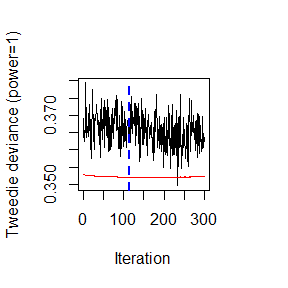optimal_perf_oob <- BT_perf(BT_algo, method='OOB', oobag.curve = TRUE, overlay = TRUE)
## OOB generally underestimates the optimal number of iterations although predictive performance is reasonably competitive.
##             Using cv_folds>1 when calling BT usually results in improved predictive performance.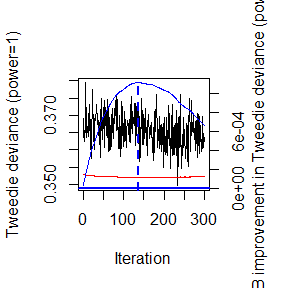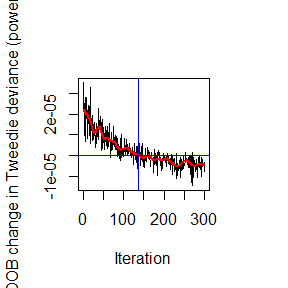optimal_perf_cv <- BT_perf(BT_algo, method='cv')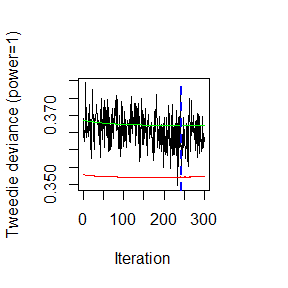optimal_perf_best_guest <- BT_perf(BT_algo, plot.it = FALSE)
## Using validation method...

### summary

This function allows to compute the relative influence and plot it. It is in fact a wrapper for the BT_relative_influence which is not intended to be used per end-user.

Up to now, the computation of the relative influence isn’t available for the permutation approach. This one should still be developed.

Regarding the currently developed method, we used the results furnished by rpart.object. In fact, each tree in the expansion is built thanks to this package and is stored in the BT$BTIndivFits list. Moreover, note that this algorithm has been adapted from rpart itself and therefore cover special cases (e.g. need to rescale for anova method). One can then present the function’s arguments: • object: a BTFit object, i.e. the result of the BT call. • n.iter: the number of iterations to use to compute the relative influence. This parameter is often set to the optimal number of iterations. By default, all the built trees will be used. • method: the function that has to be called to compute the relative influence. As previously mentioned, only one approach is currently available. This parameter should therefore remains set to its default value. • normalize: if the user wants to normalize the relative influence such that the sum over all normalized relative influence sum up to 100. • order_it: indicates whether the user wants to sort the relative influence or not. • cBars and plot_it: relative influence plot related parameters, respectively the number of bars to plot in the barplot and a boolean specifying whether the plot is expected or not. summary(BT_algo)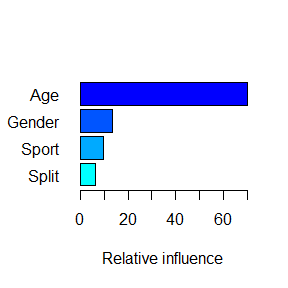## var rel_inf ## Age Age 70.162422 ## Sport Gender 13.477682 ## Gender Sport 9.855048 ## Split Split 6.504847 ### predict function Using the global fit on the training set (the unique fit if no cross-validation), one can predict (S3 method) on a new database. Here are the function arguments: • object: a BTFit object • newdata: the new data set used to realize the predictions. • n.iter: the number of boosting iterations (i.e. the number of trees) used to perform the predictions. Usually, all the iterations (i.e. the trees) up to the best one are considered to build the predictions. Please note that this parameter can be a vector. In such a case, a matrix containing the predictions for each element in n.iter will be returned. • type: Specify if one wants to predict on the ‘response’ or the ‘link’ scale. • single.iter: If set to TRUE only the n.iter tree will be used to predict (i.e. not all the trees up to n.iter). Please note that if the keep.data argument was set to TRUE and if the newdata is not specified, the prediction will be achieved on the original training set. # Predict (response scale) using all trees up to best validation iteration. head(predict(BT_algo, n.iter = optimal_perf_validation, type = 'response'), 10) ## As newdata is missing or is not a data frame, the training set has been used thanks to the keep.data = TRUE parameter. ##  0.1512079 0.1374772 0.1479626 0.1501892 0.1551795 0.1532813 0.1334080 ##  0.1412066 0.1363647 0.1244193 # Predict (link scale) using all trees up the best iteration OOB/CV. head(predict(BT_algo, newdata = dataset, n.iter = c(optimal_perf_oob, optimal_perf_cv), type = 'link'), 10)  ## [,1] [,2] ## [1,] -1.886696 -1.881140 ## [2,] -1.987535 -2.013851 ## [3,] -1.911317 -1.910444 ## [4,] -1.895761 -1.901736 ## [5,] -1.859751 -1.853420 ## [6,] -1.858016 -1.864961 ## [7,] -2.027736 -2.075813 ## [8,] -1.962980 -1.977309 ## [9,] -2.000500 -2.043417 ## [10,] -2.098513 -2.209579 # Predict using only the 40th tree. head(predict(BT_algo, n.iter = 40, type = 'response', single.iter = TRUE), 10)  ## As newdata is missing or is not a data frame, the training set has been used thanks to the keep.data = TRUE parameter. ##  1.0726613 0.7460608 1.0726613 0.9975383 1.0726613 1.0726613 0.7460608 ##  1.0726613 0.7460608 0.9109593 ### BT_more function Sometimes, it might be useful to continue the training on further iterations. This can happen e.g. if the initial n.iter parameter was not set high enough and that the best iteration computed corresponds to this value, meaning that the minimal error (and the related iteration) has yet to be found. This training continuation can be performed thanks to the BT_more function. This one has the following argument: • BTFit_object: an initial BT call on which we want to continue the training/perform more iterations. • new.n.iter: number of new boosting/tree iterations to compute. In total, the BT object will end up with n.iter + new.n.iter iterations. • is.verbose: whether or not the user wants to display algorithm evolution. • seed: optional parameter that allows reproducible example. It will then return a BTFit object (as the BT function does) augmented by the new boosting iterations. We emphasize that the call to this function call only be made if the original BT call: * has no cross-validation; * has been computed with keep.data parameter set to TRUE. We thus need to re-fit the first example (with cv.folds set to 1 and keep.data=TRUE) to show the usage of this function. BT_algo <- BT(formula = as.formula("Y_normalized ~ Age + Sport + Split + Gender"), data = dataset, ABT = F, n.iter = 300, train.fraction = 0.8, interaction.depth = 4, shrinkage = 0.01, bag.fraction = 0.5, colsample.bytree = 3, keep.data = T, is.verbose = F, cv.folds = 1, folds.id = NULL, n.cores = 1, weights = ExpoR, seed = 44) We can then call the BT_more function to perform 100 new iterations and use the new object as an usual BTFit one. BT_algo_contd <- BT_more(BT_algo, new.n.iter = 100, seed = 404) # See parameter, predict, ... BT_algo_contd$BTParams\$n.iter
##  400
head(predict(BT_algo_contd, n.iter = 350, type='link'), 10)
## As newdata is missing or is not a data frame, the training set has been used thanks to the keep.data = TRUE parameter.
##   -1.877675 -2.013065 -1.912127 -1.891241 -1.844825 -1.867253 -2.096570
##   -1.993146 -2.056822 -2.284128

## Comparison between Adaptive Boosting Tree and Boosting Tree

In our first example, we decided to fit a Boosting Tree approach. However, one can be interested in fitting an Adaptive Boosting Tree one. We note that the cross-validation is relevant for the former case while it might not be needed for the later one. In fact, by construction the Adaptive Boosting Tree will naturally ends up to root node, which will not bring more information. This is then a natural stopping criterion.

This task can easily be achieved taking the same example as before and setting ABT to TRUE instead of FALSE. It is then left to the interested reader.